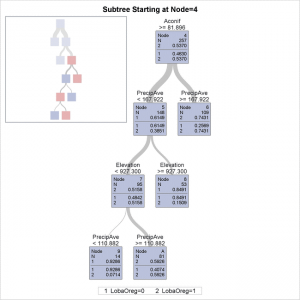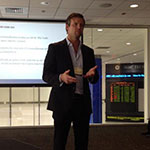As an economist, I started at SAS with a disadvantage when it comes to predictive modeling. After all, like most economists, I was taught how to estimate marginal effects of various programs, or treatment effects, with non-experimental data. We use a variety of identification assumptions and quasi-experiments to make causal interpretations, but we rarely focus on the quality of predictions. That is, we care about the “right-hand side” (RHS) of the equation. For those trained in economics in the past 20 years, predictive modeling was regarded as “data mining,” a dishonorable practice.

Since beginning my journey at SAS I have been exposed to many practical applications of predictive modeling that I believe would be valuable for economists. In this and a series of future blogs, I will write about how I think about the “left hand side” (LHS) of the equation with respect to the tools of predictive modeling. Up first: decision tree learning.Decision tree learning using the HPSPLIT procedure in SAS/STAT

Decision trees are not unfamiliar to economists. If fact, almost all economists have used trees as they learned about game theory. Game theory courses use trees to illustrate sequential games, that is, where one agent moves first. Once such as example is Stackelberg competition in which firms sequentially compete in quantities with the first mover earning greater profits. We use trees to understand sequence of decisions. The use of trees in data analysis has many similarities but some important differences. First, what are decision trees?

Decision tree learning is very simple data categorization tool that can also happens to have great predictive power. How do they work? My colleague Barry de Ville and SAS author provides a nice introduction to basics of decision tree algorithms. If you think about what these algorithms actually do, they are trying to separate data into homogenous clusters, where each cluster has highly similar explanatory covariates and similar outcomes. You can find a great guide to all many of those algorithms and more in my colleague Padraic Neville’s primer on decision trees.

So why do trees work for prediction? They subset data into observations that are highly similar on a number of dimensions. The algorithms choose to use certain explanatory factors (X’s, covariates, features), as well as interactions of those factors, to create homogeneous groups. At that point, the prediction equation is derived by some pretty complicated math……a simple average!

That’s right, once the data set is broken down into subsets (a process known as ‘splitting’ and ‘pruning’) the fancy prediction math is nothing but a simple average. And the prediction equation. Equally simple. It is a series of if-then statements following the path of the tree eventually leading to that calculated sample average. So what does the decision tree help me to do as an economist?  Here are my top 3 things to love about a decision tree:

1. Like regression, decision tree output can be interpreted. There are no coefficients but the results read like if-then-else business rules.
2. Decision trees inform about predictive power of variables WITH concerns for redundancy. Variables will be split on if they matter for creating homogeneous groups and discarded otherwise. One caveat, however, is that only one of two highly collinear variables might be chosen.
3. They inform about interaction effects for later regression analysis. A split tells us that an interaction effect matters in prediction. This could be useful for controlling for various forms of unobserved heterogeneity or for turning continuous variables into categorical variables.

So that is my list. What else should economists know about decision trees? Do you feel strongly that trees are a better exploratory data analysis tool than predictive tool?

Next time we will augment this discussion of decision trees by talking about, What Economists should know about … random forests.

Share